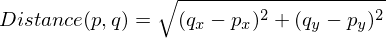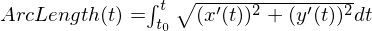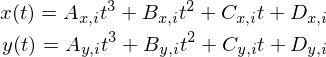Introduction to Series
This is the 3rd post on splines in a series I am writing primarily for game programmers and others interested in the basics of using splines in their software.
In part 1 of this series I wrote an introduction to cubic splines. In part 2 I showed one way for how to find the closest point on a spline path to another point in the world.

Introduction to Post
Today I will discuss the problem of finding the length of a spline. I will go into why it is useful and a little bit of the mathematics behind it. Afterwards I have some example code for an approach to finding the arc length. I also briefly discuss other approaches that may improve the accuracy of computing the length.

What is the Arc Length?
When people discuss length in the context of a spline, they usually mean the arc length. This is how long the spline would be if it were stretched out without any curves. You can also think of this as the distance you would need to travel if you walked along the spline from the beginning to end.

Why is it Useful?
When programming a game that uses splines, it is useful for many reasons to know the arc length. In a city-building game the amount of road the player places down is proportional to how much it costs the player. If the road were represented as spline paths, then the arc length would be used to determine the total cost. In a racing game, the arc length could represent the length of the track if the programmer had chosen to represent the track as a spline path.

How to Specify it Mathematically
The ordinary distance between two points p and q in 2D is:We can approximate the arc length of the spline by finding points along the spline, and adding up all of the lengths between each consecutive point. If we subdivide the spline path into smaller and smaller segments, then the approximation more closely matches the true arc length. To say it another way, the limit of the sum of lengths between consecutive points, as the number of points approaches infinity, is the arc length.

Mathematically this is represented as an integral over the spline. It can be written like this:The functions x and y are the dimensional functions for the spline in 2D. The variable t is the spline parameter. For a cubic spline, these functions are written below:A_xi is the first spline coefficient in the x dimension on spline segment i.

Code Example
The simplest way to find the arc length for a spline in code is to choose a number of points along the spline, and add up the lengths between each consecutive point. One way to write this code:
  1 2 3 4 5 6 7 8 9 10 11 12 13 14 15 16 17 18 19 20 21 22 23 24 25 26 27 28 29 30 31 32 33 34 35 36 37 38 39 40 vec2 SplineSegment(vec2 a, vec2 b, vec2 c, vec2 d, float x) { vec2 result; // ax^3 + bx^2 + cx + d result.x = x*(x*(a.x*x + b.x) + c.x) + d.x; result.y = x*(x*(a.y*x + b.y) + c.y) + d.y; return result; } float SplineTotalArcLength(const cubic_spline *spline) { float totalLength = 0.0f; for(int segment = 0; segment < spline->controlPointCount; segment++) { const float granularity = 16.0f; // Integration samples per spline segment. vec2 last = spline->coefficients[segment]; float segmentLength = 0.0f; for(float i = 1.0f; i <= granularity; i += 1.0f) { float x = i / granularity; vec2 next = SplineSegment(spline->coefficients[segment], spline->coefficients[segment], spline->coefficients[segment], spline->coefficients[segment], x); float subsegmentLength = Length(next - last); last = next; segmentLength += subsegmentLength; } totalLength += segmentLength; } return totalLength; } 

Other Techniques
This solves the problem of finding the arc length, but it is not necessary the most accurate algorithm to do the job. While it will still closely approximate the arc length of the spline if the number of points is sufficiently large, there are other methods that give more accurate results with the same number of control points

Since we have framed this problem as an integral over the spline path, we can use other conventional numerical analysis techniques to solve the integral. These other approaches are beyond the scope of this article. For those interested, the techniques to look up are Simpson’s Rule or Gaussian quadrature.

Conclusion
I showed how finding the arc length is applicable to many challenges game programmers may face when working with splines, as well as a straightforward approach to finding the arc length. In the next article I will discuss a technique for getting smooth constant velocity movement along a spline path without relying on numerical analysis techniques that need to run in real-time.